### Home > CALC > Chapter Ch2 > Lesson 2.3.2 > Problem2-115

2-115.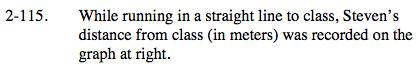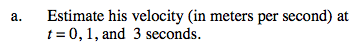Velocity is the steepness (or slope) of a distance graph. Since this graph is not linear, slope can only be approximated.

Think about ramp lab (2-112). Slope at a specific point was approximated by finding the slope of a line that connects two points close to the target.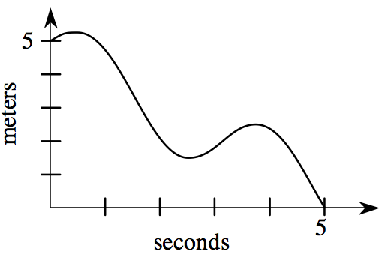We can approximate the slope at t = 1 by choosing two points close to t = 1 and finding the slope of the line that connects them.

$\text{velocity at } t = 1 \text{ is about } -\frac{5}{2} \text{ m/sec}$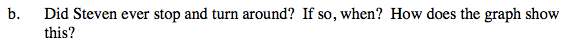This is a distance graph. y-values represent distance from the classroom.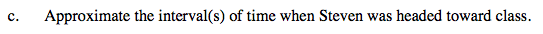The classroom is located at y = 0.